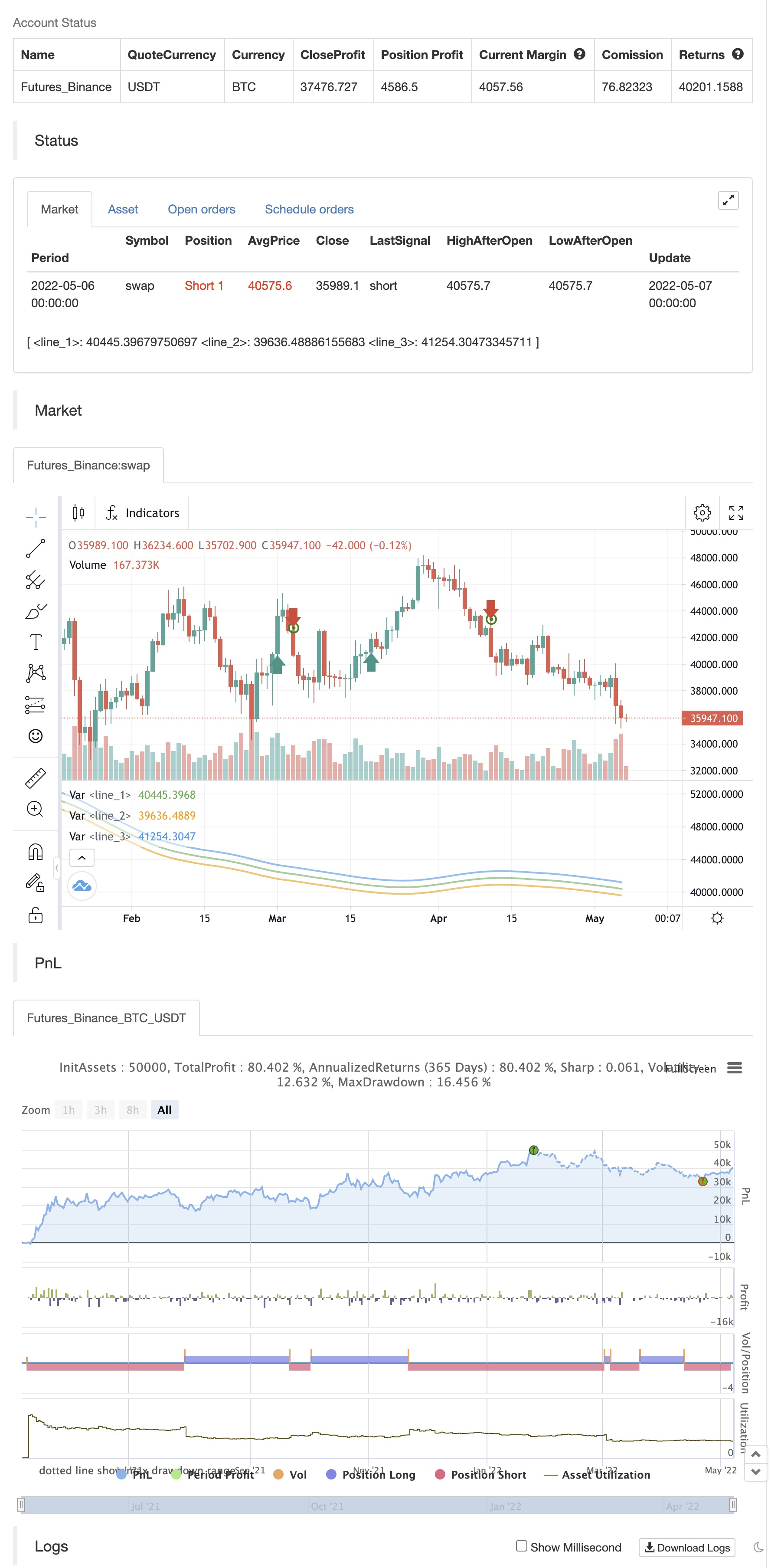# EHMA Range Strategy

Author: 张超, Date: 2022-05-09 23:31:59
Tags: EHMA

This script is a modified version of @borserman’s script for the Exponential Hull Moving Average All credit for the EHMA goes to him :)

In addition to the EHMA, this script works with a range around the EHMA (which can be modified), in an attempt to be robust against fake signals. Many times a bar will close below a moving average, only to reverse again the next bar, which eats away at your profits. Especially on shorter timeframes, but also on choppy longer timeframes this can make a strategy unattractive to use.

With the range around the EHMA, the strategy only enters a long/exit-short position if a bar crosses above the upper range. Vice versa, it only enters a short/exit-long position if a bar crosses below the lower range. This avoids positions if bars behave choppy within the EHMA range & only enters a position if the market is confident in it’s direction. Having said that, fakeouts are still possible, but a lot less frequent. Having backtested this strategy vs the regular EHMA strategy (and having experimented with various settings), this version seems to be a lot more robust & profitable!

Disclaimer Please remember that past performance may not be indicative of future results. Due to various factors, including changing market conditions, the strategy may no longer perform as good as in historical backtesting. This post and the script don’t provide any financial advice.

backtest```/*backtest
start: 2021-05-08 00:00:00
end: 2022-05-07 23:59:00
period: 1d
basePeriod: 1h
exchanges: [{"eid":"Futures_Binance","currency":"BTC_USDT"}]
*/

// Credit is due where credit is due:
// Hull Moving Average: developed by Alan Hull
// EHMA: coded by Twitter @borserman
// I've built on their work in an attempt to create a strategy more robust to fake moves
// @0xLetoII

//@version=4
//strategy(
//  title="EHMA Range Strategy",
//  process_orders_on_close=true,
//  explicit_plot_zorder=true,
//  overlay=true,
//  initial_capital=1500,
//  default_qty_type=strategy.percent_of_equity,
//  commission_type=strategy.commission.percent,
//  commission_value=0.085,
//  default_qty_value=100
//  )

// Position Type
pos_type = input(defval = "Both", title="Position Type", options=["Both", "Long", "Short"])

// Inputs
Period = input(defval=180, title="Length")
RangeWidth = input(defval=0.02, step=0.01, title="Range Width")
sqrtPeriod = sqrt(Period)

// Function for the Borserman EMA
borserman_ema(x, y) =>
alpha = 2 / (y + 1)
sum = 0.0
sum := alpha * x + (1 - alpha) * nz(sum)

// Calculate the Exponential Hull Moving Average
EHMA = borserman_ema(2 * borserman_ema(close, Period / 2) - borserman_ema(close, Period), sqrtPeriod)

// Create upper & lower bounds around the EHMA for broader entries & exits
upper = EHMA + (EHMA * RangeWidth)
lower = EHMA - (EHMA * RangeWidth)

// Plots
EHMAcolor = (close > EHMA ? color.green : color.red)
plot(EHMA, color=EHMAcolor, linewidth=2)
plot(lower, color=color.orange, linewidth=2)
plot(upper, color=color.blue, linewidth=2)

// Strategy
long = close > upper
exit_long = close < lower
short = close < lower
exit_short = close > upper

// Calculate start/end date and time condition
//startDate  = input(timestamp("2017-01-01T00:00:00"))
//finishDate = input(timestamp("2029-01-01T00:00:00"))

time_cond  = true

// Entries & Exits
if pos_type == "Both"
strategy.entry("Long", strategy.long, comment="Long", when=long and time_cond)
strategy.close("Long", comment="Exit Long", when=exit_long and time_cond)
strategy.entry("Short", strategy.short, comment="Short", when=short and time_cond)
strategy.close("Short", comment="Exit Short", when=exit_short and time_cond)
if pos_type == "Long"
strategy.entry("Long", strategy.long, comment="Long", when=long and time_cond)
strategy.close("Long", comment="Exit Long", when=exit_long and time_cond)
if pos_type == "Short"
strategy.entry("Short", strategy.short, comment="Short", when=short and time_cond)
strategy.close("Short", comment="Exit Short", when=exit_short and time_cond)

```

Related

More